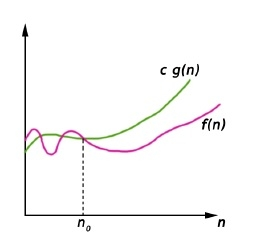# Big Oh Notation (O)

## Asymptotic Notations

Asymptotic notations are used to represent the complexities of algorithms for asymptotic analysis. These notations are mathematical tools to represent the complexities. There are three notations that are commonly used.

## Big Oh Notation

Big-Oh (O) notation gives an upper bound for a function f(n) to within a constant factor.

We write f(n) = O(g(n)), If there are positive constants n0 and c such that, to the right of n0 the f(n) always lies on or below c*g(n).

O(g(n)) = { f(n) : There exist positive constant c and n0 such that 0 ≤ f(n) ≤ c g(n), for all n ≤ n0}